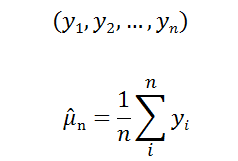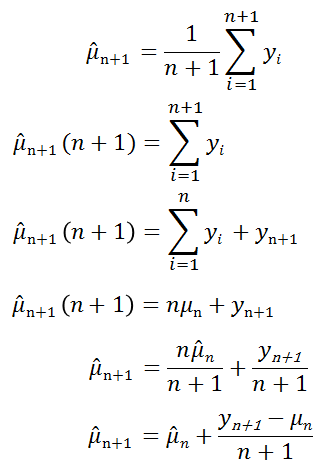# ICRFS™ algorithms for model estimation, forecasts, and simulations: why are they so fast?

The algorithms in ICRFS™ for estimating models, forecasting log-normal distributions and their sum, and simulating from the log-normal distributions have been heavily optimised over many years. The primary drivers of optimisations are a result of a fundamental understanding of the mathematics specifying the Probabilistic Trend Family (PTF) and Multiple Probabilistic Trend Family (MPTF) models. Wherever possible, calculations are done once.

For instance, consider the problem when the sample mean (size n) is calculated. The formula for the sample mean is:Now consider we have a new observation yn+1, how can we calculate the new sample mean?

The simple solution is to sum up the numbers and divide by the size of the sample (first line below), however this does not take into account the information obtained from the previous calculation: the sample mean of the data to n is already known.

The update to the sample mean (mu) can be simplified to:The final calculation is an update of the previous value of the sample mean - that is, the formula is recursive.

For large values of n, the saving in both storage space and calculation time is significant. The concept of updating existing calculations based on new data is not new. In fact, the Kalman Filter formed the starting point for developing fast algorithms; particularly for MPTF where numerous statistics are estimated. Really fast update algorithms for estimation and forecasting can be developed if you take very careful account of the details of the special structure of the model classes being used.

Now consider the yn's as vectors, the recursive formulation provides a huge computational advantage as the same principle applies when more than one sample element is added. For example, a vector of new data arrives; a calendar year's paid losses. When trend parameters, correlations, and volatility are updated, Insureware's algorithms are designed in such a way that minimal recalculations of previous data are required.

Articles in this category
• Technical

(c) Insureware 1985 ~ 2021

Insureware Pty. Ltd.
info@insureware.com